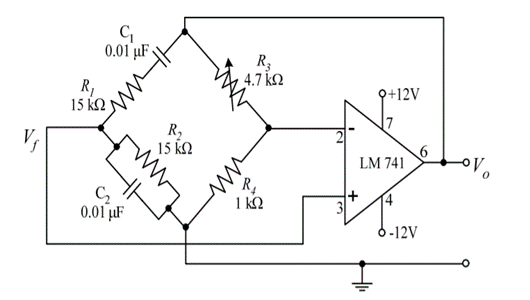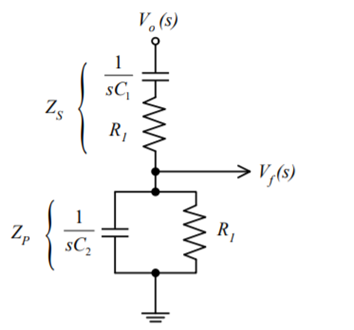Goseeko blog# What is the Wein bridge oscillator?

The Wien bridge oscillator is an audio frequency sine wave oscillator of high stability and simplicity. The non-inverting input terminal in the circuit is connected to the feedback signal and hence the op-amp works as a non-inverting amplifier.

## Operation of Wein bridge oscillator

In the Wein bridge oscillator circuit the feedback network has no phase shift. When circuit elements R1 and C1 are connected in series of one bridge arm and R2 and C2 in parallel combination to the adjoining arm. The other two arms of the bridge are connected to resistors R3 and R4.

The condition of zero phase shift is achieved when the bridge is balanced. The lead-lag circuit is then formed by the series and parallel combination of RC networks.

Then reactance of capacitor C1 and C2 approaches zero at high frequencies. Which then causes C1 and C2 to appear short. Due to the capacitor C2 the resistor R2 is short circuited. As the output is taken across R2 and C2 then output voltage Vo is zero. The circuit now acts as a lag circuit at high frequencies.

At low frequencies, both capacitors act as open because the capacitor offers very high reactance. Again, output voltage will be zero because the input signal is d across the R1 and C1 combination. Here, the circuit acts like a ‘lead circuit’.

The output voltage also reaches the maximum value at one particular frequency between the two extremes. We obtain maximum output at this frequency only when the resistance value is equal to the capacitive reactance and gives maximum output. This frequency is the oscillating frequency represented by f.## Derivation

Applying voltage divider rule in above circuit we get

Vf(s) = Vo(s) x Zp(s)/ Zp(s) + Zs(s)

Zs(s) = R1 + 1/sC1 and Zp(s) = R2|| 1/sC2

Let R1=R2=R and C1=C2=C . On solving

β = Vf(s)/ Vo(s) = RsC /(RsC) 2 + 3RsC + 1 —————————(1)

The voltage gain of op-amp will now becomes

Av = Vo(s)/ Vf(s) = 1 + R3/R4 ——————-(2)

Applying the condition for sustained oscillations, = Av β =1

RsC /(RsC) 2 + 3RsC + 1. 1 + R3/R4

S=j⍵

(1 + R3/R4) ( j⍵RC/ – R2 C22 + 3 j⍵RC + 1) =1

j⍵ RC (1 + R3/R4)= (- R2 C22 + 3 j⍵RC + 1)

j⍵[(1 + R3/R4)RC – 3RC] = 1- R2 C22

Equating real part to zero and finding frequency of oscillations

1-     R2 C22   = 0

⍵ = 1/RC

f = 1/ 2 π RC

We equate the imaginary part to zero for finding the condition for gain at oscillating frequency.

j⍵[(1 + R3/R4)RC – 3RC] = 0

j⍵[(1 + R3/R4)RC= j⍵3RC

[(1 + R3/R4) =3

R3/R4 =2

Therefore R3 = 2 R4 is the required condition.

Interested in learning about similar topics? Here are a few hand-picked blogs for you!